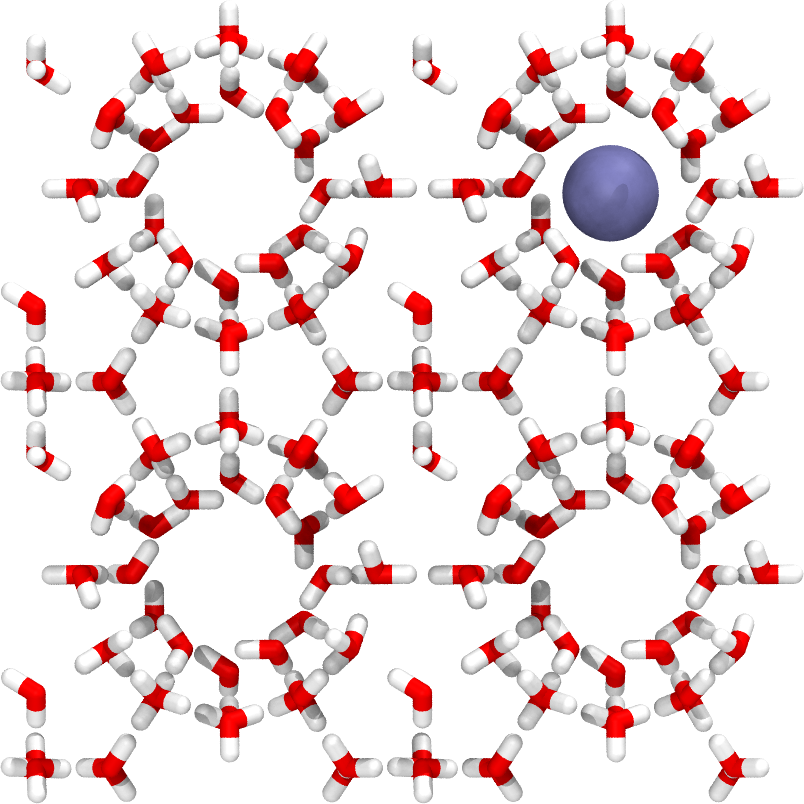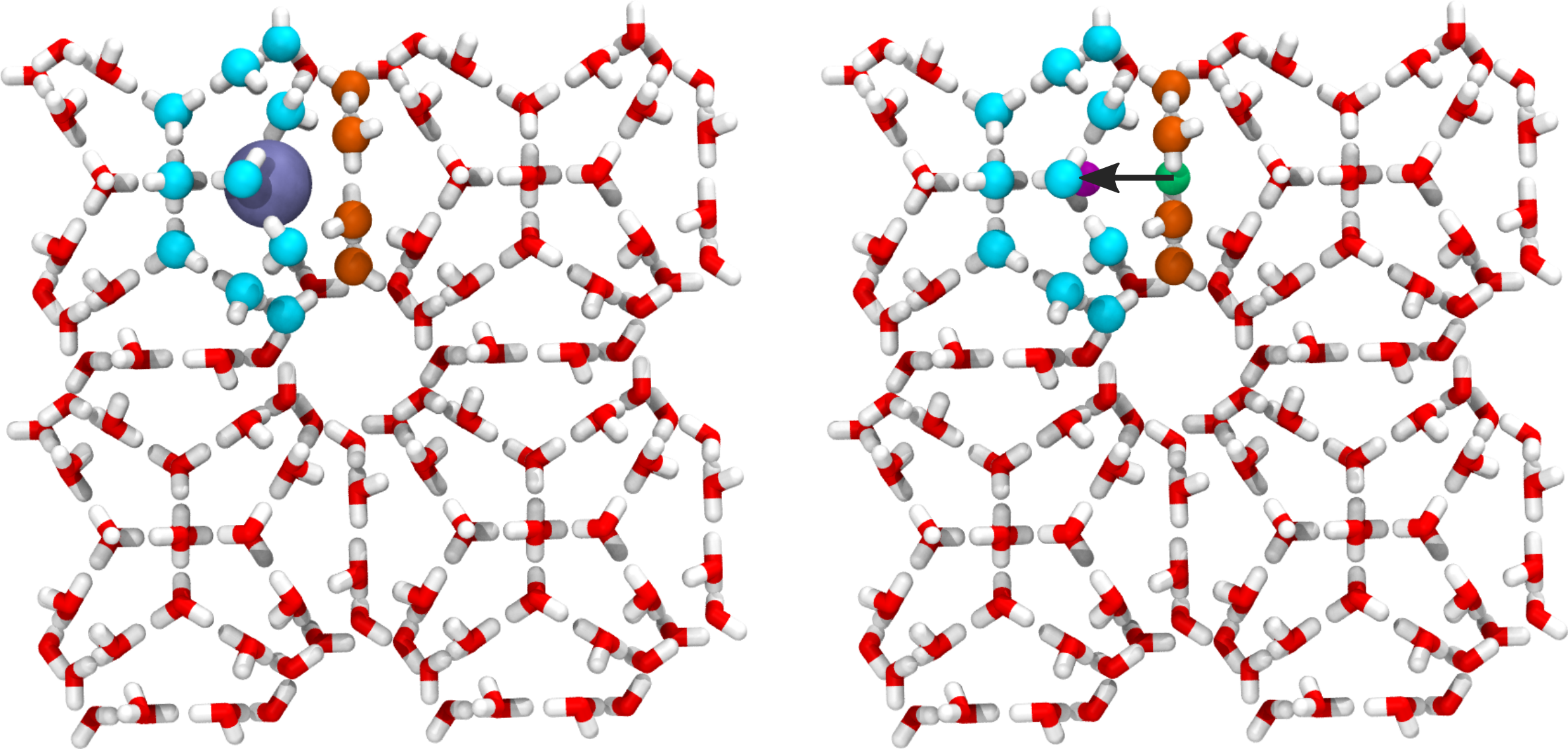# Transport of methane in a sI hydrate¶

In this example, we are going to study the transport of methane in a sI hydrate structure. The initial configuration is shown in Fig. 38.Fig. 38 A snapshot of the sI hydrate system. The methane molecule is modeled as a single particle (united atom) and is here shown as a blue sphere.

The process we will investigate is the diffusion of the methane molecule between different cages in the hydrate structure. The order parameter which measures the progress of the methane molecule is defined as the distance traveled by the methane molecule along a vector pointing between the geometric center of selected rings in the hydrate structure (see Fig. 39 for an illustration).

In this example, we will make use of GROMACS  for running the dynamics. The example is structured as follows:

## Defining the order parameter¶

The order parameter we make use of is illustrated below.Fig. 39 Illustration of the order parameter used for the hydrate example. Oxygen atoms in two cages/rings are identified (colored cyan and orange) and the geometric center of these two groups is obtained (shown as the magenta and green spheres in the right image). This is used to define a vector between the two centers (illustrated with the black arrow in the right image). The order parameter is taken as the distance vector between the methane and the first center (green sphere) projected onto the vector joining the two centers.

This is a custom order parameter and we will now create a Python script for calculating it. We refer to the section on creating custom order parameters for more detailed information on how this can be done. Here, we just give the full Python script for calculating the order parameter:

```# -*- coding: utf-8 -*-
# Copyright (c) 2021, PyRETIS Development Team.
# Distributed under the LGPLv2.1+ License. See LICENSE for more info.
"""This file defines the order parameter used for the hydrate example."""
import logging
import numpy as np
from pyretis.orderparameter import OrderParameter
logger = logging.getLogger(__name__)  # pylint: disable=invalid-name
logger.addHandler(logging.NullHandler())

class RingDiffusion(OrderParameter):
"""RingDiffusion(OrderParameter).

This class defines the ring diffusion order parameter for
the hydrate example.

Attributes
----------
name : string
A human readable name for the order parameter
index : integer
This selects the particle to use for the order parameter.
periodic : boolean
This determines if periodic boundaries should be applied to
the position or not.

"""

def __init__(self):
"""Initialise the order parameter.

Parameters
----------
name : string
The name for the order parameter
index : tuple of ints
This is the indices of the atom we will use the position of.
periodic : boolean, optional
This determines if periodic boundary conditions should be
applied to the position.

"""
super().__init__(description='Ring diffusion for hydrate')
self.idx1 = np.array([56, 64, 104, 112, 200, 208], dtype=np.int16)
# convert to atom index:
self.idx1 *= 4
self.idx1 -= 3
# convert to 0 index:
self.idx1 -= 1
self.idx2 = np.array([56, 64, 72, 80, 104, 112, 136, 152, 168, 176,
200, 208, 232, 248, 264, 272, 296, 304, 328,
336, 344, 352, 360, 368], dtype=np.int16)
# convert to atom index:
self.idx2 *= 4
self.idx2 -= 3
# convert to 0 index:
self.idx2 -= 1
self.idxd = 1472  # index for diffusing atom

def calculate(self, system):
"""Calculate the order parameter.

Here, the order parameter is just the distance between two
particles.

Parameters
----------
system : object like :py:class:`.System`
This object is used for the actual calculation, typically
only `system.particles.pos` and/or `system.particles.vel`
will be used. In some cases `system.forcefield` can also be
used to include specific energies for the order parameter.

Returns
-------
out : float
The order parameter.

"""
pos = system.particles.pos
resl = 1.0e3
cm1 = np.average(np.rint(pos[self.idx1] * resl) / resl, axis=0)
cm2 = np.average(np.rint(pos[self.idx2] * resl) / resl, axis=0)
cmvec = cm2 - cm1
molvec = np.rint(pos[self.idxd] * resl) / resl
molvec -= cm1
orderp = -np.dot(cmvec, molvec) / np.sqrt(np.dot(cmvec, cmvec))
return [orderp]

if __name__ == '__main__':
testo = RingDiffusion()
print('Idx1', testo.idx1)
print('Idx2', testo.idx2)
```

This order parameter can be used in a simulation in PyRETIS by adding the following order parameter section to the input file:

```Orderparameter
--------------
class = RingDiffusion
module = orderp.py
```

In addition, we can add extra order parameters, for instance the position (z-coordinate) of the diffusing methane molecule:

```Collective-variable
-------------------
class = Position
index = 1472  # This is the methane molecule
dim = z
```

## Creating the PyRETIS input file¶

The input file for PyRETIS follows the structure we have used in the previous examples and we will not go into details about all sections here. In the following, we will just describe the section for using the GROMACS engine in more detail. The full input file for the RETIS simulation is given at the end of this section.

In order to make use of GROMACS, we add the following engine section to the input file:

```Engine settings
---------------
class = gromacs
gmx = gmx_2016.4
mdrun = gmx_2016.4 mdrun
input_path = gromacs_input
timestep = 0.002
subcycles = 5
```

Here, we make use of the following keywords:

• `class = gromacs` which specifies the engine we will use (here: gromacs).

• `gmx = gmx_5.1.4` which specifies the GROMACS gmx executable. On your system, this might be named differently, for instance `gmx = gmx`.

• `mdrun = gmx_5.1.4 mdrun` which specifies the command for running GROMACS. Note that you here can tune how to run GROMACS, for instance by adding a `mpirun` or something similar if that fits your system.

• `input_path = gromacs_input` which specifies the directory where PyRETIS will look for the input files for GROMACS. PyRETIS assumes that, at least, the following files are in this folder:

1. `conf.g96` which contains the initial configuration to use.
2. `topol.top` which contains the topology for GROMACS.
3. `grompp.mdp` which contains simulation settings for GROMACS.

The files needed for this example can be downloaded as a zip archive here: `gromacs_input.zip`.

• `timestep = 0.002` which is the time step to use in the GROMACS simulations.

• `subcycles = 5` which is the number of subcycles GROMACS will complete before PyRETIS re-calculated order parameter. This will also be the frequency by which GROMACS write information (trajectory and energies) to the disk.

This specifies and selects the GROMACS engine for use with PyRETIS. The full input file for the RETIS simulation is given below:

```Retis 1D example
================

Simulation
----------
task = retis
steps = 10
interfaces = [-0.26, -0.24, -0.22, -0.20, -0.19, -0.18, -0.17,
-0.16, -0.15, -0.14, -0.13, -0.12, -0.11, -0.10,
-0.09, -0.08, -0.07, -0.06, -0.05, -0.04, -0.02,
0.00,  0.02,  0.20]

System
------
units = gromacs

Engine settings
---------------
class = gromacs
gmx = gmx_2016.4
mdrun = gmx_2016.4 mdrun
input_path = gromacs_input
timestep = 0.002
subcycles = 5
gmx_format = g96

TIS settings
------------
freq = 0.5
maxlength = 20000
aimless = True
allowmaxlength = False
zero_momentum = False
rescale_energy = False
sigma_v = -1
seed = 0

RETIS settings
--------------
swapfreq = 0.5
relative_shoots = None
nullmoves = True
swapsimul = True

Initial-path
------------
method = kick
kick-from = previous

Orderparameter
--------------
class = RingDiffusion
module = orderp.py

Collective-variable
-------------------
class = Position
index = 1472  # This is the methane molecule
dim = z

Output
------
order-file = 1
```

## Running the RETIS simulation with PyRETIS¶

In order to run the RETIS simulation, the following steps are suggested:

1. Create a new folder for the simulation named `gromacs-hydrate` and enter this directory.

2. Create a new file `orderp.py` containing the script for calculating the order parameter.

3. Create a new file `retis.rst` with the input settings for the simulation.

4. Download the input files (`gromacs_input.zip`) for GROMACS and store these in a new directory named `gromacs_input`.

5. Run the RETIS simulation using:

```pyretisrun -i retis.rst -p
```

This will execute the PyRETIS simulation. An example of a reactive trajectory (obtained in the last path ensemble) is shown in Fig. 40.Fig. 40 Snapshots from a trajectory in the last path ensemble. The methane molecule (colored blue) exits from the starting cage and enter the next cage.